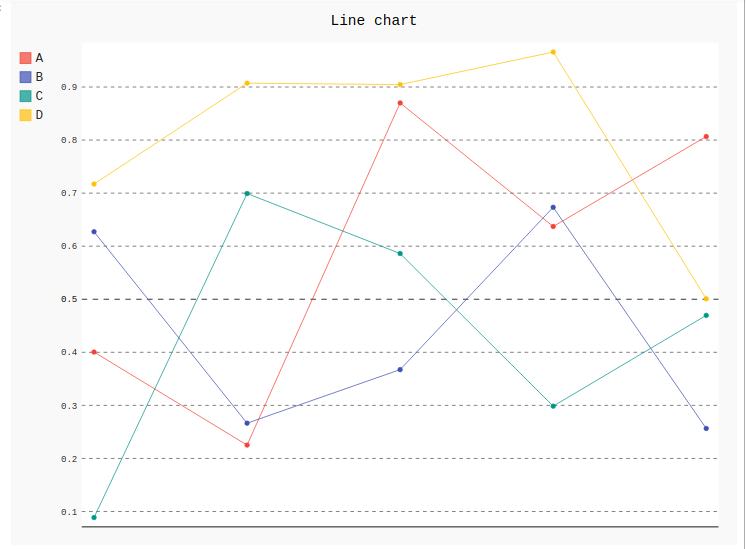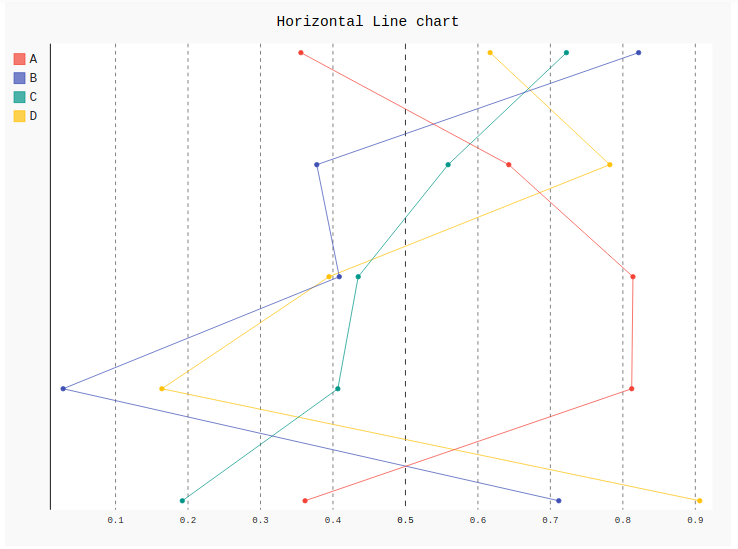# Line chart in Pygal

A line chart or line graph is a type of chart which helps to displays the information as a series of all data points called ‘markers’ and those markers are connected straight through line segments. It is a basic type of chart common in many fields which shows ascending or descending in a graph. A line chart is often used to visualize a trend in data over intervals of time which helps to represent the graph more effectively. Dimensions in the data are often displayed on the x-axis and y-axis.

## Creating Line Chart

1) Simple Line Chart: Simple basic line graphs are used for showing the relationship between two variables in vertical form. Line() method is used to create simple line graphs in Pygal.

Syntax:

`line_chart = pygal.Line()`

Example:

 `# importing pygal ` `import` `pygal ` `import` `numpy ` ` `  ` `  `# creating line chart object ` `line_chart ``=` `pygal.Line() ` ` `  `# naming the title ` `line_chart.title ``=` `'Line chart'` ` `  ` `  `# adding lines ` `line_chart.add(``'A'``, numpy.random.rand(``5``)) ` `line_chart.add(``'B'``, numpy.random.rand(``5``)) ` `line_chart.add(``'C'``, numpy.random.rand(``5``)) ` `line_chart.add(``'D'``, numpy.random.rand(``5``)) ` ` `  `line_chart `

Output:2) Horizontal line chart: Horizontal line graph is the same as a basic line graph but the difference is it shows line horizontally from x-axis to y-axis. Both the variables are shown horizontally.

Syntax:

`line_chart = pygal.HorizontalLine()`

Example:

 `# importing pygal ` `import` `pygal ` `import` `numpy ` ` `  ` `  `# creating line chart object ` `line_chart ``=` `pygal.HorizontalLine() ` ` `  `# naming the title ` `line_chart.title ``=` `'Horizontal Line chart'` ` `  ` `  `# adding lines ` `line_chart.add(``'A'``, numpy.random.rand(``5``)) ` `line_chart.add(``'B'``, numpy.random.rand(``5``)) ` `line_chart.add(``'C'``, numpy.random.rand(``5``)) ` `line_chart.add(``'D'``, numpy.random.rand(``5``)) ` ` `  `line_chart `

Output:My Personal Notes arrow_drop_upIf you like GeeksforGeeks and would like to contribute, you can also write an article using contribute.geeksforgeeks.org or mail your article to contribute@geeksforgeeks.org. See your article appearing on the GeeksforGeeks main page and help other Geeks.

Please Improve this article if you find anything incorrect by clicking on the "Improve Article" button below.

Article Tags :

Be the First to upvote.

Please write to us at contribute@geeksforgeeks.org to report any issue with the above content.